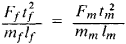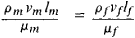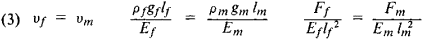# Modeling, Physical

The following article is from The Great Soviet Encyclopedia (1979). It might be outdated or ideologically biased.

## Modeling, Physical

a type of modeling in which the study of an object or phenomenon is replaced by the experimental study of its model, which is of the same physical nature.

Any scientific experiment that is performed in order to reveal specific principles of a phenomenon or to check the accuracy and useful limits of theoretically deduced results is essentially modeling, because the object of the experiment is a specific model that has the necessary physical properties and that must meet the fundamental requirements imposed on physical modeling during the experiment. Physical modeling is used in technology in the design and construction of a variety of objects in order to determine—on the corresponding models—some particular properties (characteristics) of the object as a whole and of its separate parts. Physical modeling is used not only because of economic considerations but also because the performance of full-scale tests is very difficult or totally impossible when the dimensions of the full-scale object or the values of its other characteristics, such as the pressure, temperature, or rate of the process, are too large or small.

Physical modeling is based on similarity theory and dimensional analysis. The conditions required are geometric similarity (similarity of shape) and physical similarity between the model and the original: at similar moments and similar points in space, the values of the variables that describe the full-scale phenomena must be proportional to the values of the same quantities for the model. The existence of such proportionality makes possible translation to full scale of the results obtained experimentally on the model by multiplying each of the quantities measured by the similarity factor, which is constant for all values of a given unit of measurement.

Since physical quantities are associated by definite relationships that follow from the laws and equations of physics, by choosing certain of them as basic it is possible to express the similarity factors for all the other derived quantities in terms of the similarity factors for those chosen as basic. For example, in mechanics the quantities usually assumed to be basic are length l, time t, and mass m. Then, since velocity v = l/t, the similarity factor for velocity kv = vf/vm (the subscripts f and m on the quantities represent the full-scale object and the model, respectively) can be expressed in terms of the similarity factors for length (kl = lf/lm) and time (kt = tf/tm), so that kv = kl/kt. Correspondingly, because force F is related to acceleration w by the expression F = mw, on the basis of Newton’s second law, then Kf = km · kw, where, in turn, kw = kv/kt, and so on. From the existence of such relationships it follows that, for a given physical phenomenon, certain dimensionless combinations of quantities that describe the phenomenon must have the same value for the model and the original. These dimensionless combinations of physical quantities are called similarity criteria. The equality of all similarity criteria for a model and the original is a necessary condition for physical modeling. However, it is not always possible to secure this equality, because not all the similarity criteria can always be satisfied at the same time.

Physical modeling is most often used in the study of various thermal, electrical, and mechanical phenomena (including phenomena in aerohydromechanics and the mechanics of deformable solids). Here the number and type of similarity criteria for each of the phenomena being modeled depend on its nature and characteristics. Thus, as an example, for problems of the dynamics of a point (or a system of point masses), in which all the equations follow from Newton’s second law, the similarity criterion is the Newton number Ne = Ft2/ml, and the condition for modeling is

(1) Net = Nem

that is,For the oscillations of a weight when acted upon by an elastic force F = cl equation (1) gives the condition tf2 Cf/mf = tm2cm/mm, which, as an example, makes it possible to determine the period of oscillation of the original from the period of oscillation of the model; in this case the phenomenon does not depend on the linear scale (the oscillation amplitude). For motion in a gravitational field, where F = km/l2, the similarity condition is kftf2/lf3 = kmtm2/lm3 (the phenomenon does not depend on mass). For motion in a single gravitational field, such as that of the sun, km = kf, and the relationship obtained gives Kepler’s third law for the orbital period. Hence, by taking one of the planets as a “model,” the orbital period of any other planet can be found if its distance from the sun is known.

In the study of the motion of a continuous medium, the number of similarity criteria becomes larger, thus frequently causing substantial complication of the problem of physical modeling. The basic similarity criteria in aerohydrodynamics are the Reynolds number Re, the Mach number M, the Froude number Fr, the Euler number Eu, and, for transient (time-dependent) flows, the Strouhal number St as well. For the physical modeling of phenomena that are associated with heat transfer in moving fluids and gases or with physicochemical transformations of the components in gaseous flows, it is necessary to take into account a number of additional similarity criteria.

The experimental apparatus and models created for aerohydrodynamic modeling must provide equality of the corresponding criteria for the model and the original. This can usually be accomplished when only one of the similarity criteria for the flow is retained because of its special characteristics. Thus, for physical modeling of the steady flow of an incompressible viscous fluid (gas), the determining parameter will be Re, and it is necessary to satisfy one condition:

(2) Rem = Ref

that is,where ρ is the density of the medium, and μ is its dynamic viscosity coefficient. For a scale model (lm < lf), this can be done either by increasing the velocity (vm > vf) or by using another fluid for the modeling such that, for example, ρm > ρf and μm ≦ μf. In aerodynamic studies it is impossible to increase vm in this case (the incompressibility condition is violated), but ρm can be increased by using wind tunnels of the closed type, in which compressed air is circulated.

When equality of several criteria must be provided in physical modeling, serious difficulties arise that are often insuperable unless the model becomes identical with the original, which means a transition from physical modeling to full-scale testing. Consequently approximate modeling is often used in practice; in this case those processes that play a secondary role are modeled either not at all or only approximately. This kind of physical modeling does not permit determination by direct calculation of those characteristics that do not meet the similarity conditions; their determination necessitates additional research. For example, in physical modeling of the steady flows of viscous compressible gases, it is necessary to provide equality of the criteria Re and M and the dimensionless number K = Cp/Cv (Cp and Cv are the specific heats of the gas at constant pressure and constant volume, respectively), which is usually impossible. Therefore, as a rule, only equality of the number M is provided for the model and the original, and the effect of differences in the numbers Re and K on the parameters being determined is studied separately, either theoretically or through other experiments in which the values of Re and K: are varied over a fairly wide range.

For deformable solids the characteristics of physical modeling also depend on the properties of the bodies studied and on the nature of the problems being considered. Thus, when modeling the equilibrium of uniform elastic systems (structures) in which the mechanical properties depend on the elastic modulus (Young’s modulus) E and the dimensionless Poisson ratio v, three similarity conditions must be met:where g is the acceleration of gravity (γ = ρg is the specific weight of the material). Under natural conditions gm = gf = g, and it is possible to achieve complete similarity when lm = ≠ lf only by choosing special material for the model such that ρm, Em, and vm will meet the first two of the conditions in (3), which is usually not feasible in practice.

One of the types of physical modeling used on solid deformable solids is the optical-polarization method of studying stresses, which is based on the ability of a number of isotropic transparent materials to become anisotropic upon loading (that is, upon deformation), thereby making it possible to investigate the stress distribution in various parts by means of models made of such transparent materials.

For physical modeling of the phenomena in other continuous media, there are corresponding changes in the kind and number of similarity criteria. Thus, for plastic and viscoplastic media, the criteria include the Lagrange, St. Venant, and Stokes numbers, along with the Froude and Strouhal numbers and a modification of the Reynolds number.

Physical modeling is also used extensively in the study of heat-transfer processes. For the case of heat transfer by convection, the controlling similarity criteria are the Nusselt number Nu = αl/λ, the Prandtl number Pr = v/α, the Grashof number Gr = βgl3 Δ T/v2, and the Reynolds number Re, where α is the heat transfer coefficient, α is the coefficient of thermal diffusivity, λ is the coefficient of thermal conductivity of the medium (fluid or gas), v is the kinematic viscosity coefficient, β is the coefficient of volumetric expansion, and ΔT is the temperature difference between the surface of the body and the medium. The objective of the physical modeling is usually the determination of the heat-transfer coefficient, which is part of the Nu criterion and for which tests on models establish the dependence of Nu on the other criteria. Moreover, in the case of forced convection (such as heat transfer during the movement of a fluid in a pipe), the criterion Gr becomes unimportant, and in the case of free convection (heat transfer between a body and a quiescent medium) the criterion Re also becomes unimportant. However, this does not lead to substantial simplification of the physical modeling process, particularly because of the criterion Pr, which is a physical constant of the medium and, when the condition Prm = Prf is met, virtually eliminates the possibility of using for the model a medium other than the actual medium. Additional difficulties are introduced by the temperature dependence of the medium’s physical characteristics. Consequently, in most cases of practical importance all the similarity conditions cannot be met; approximate modeling must be used. Here the equality condition of the criteria that have little influence on the process is abandoned, and the other conditions (for example,” the similarity of the physical properties of the media that take part in the heat transfer) are satisfied only on the average. The “local” thermal modeling method, which is based on the idea that the similarity conditions of the processes for the model and the original are met only in the area of the model where the heat-transfer process is under study, is also used in practice. For example, when studying heat transfer in a system of bodies of the same type (spheres or pipes), only one body—on which measurements are made—may participate in the heat exchange, and the others are used to provide geometric similarity between the model and the original.

In the case of heat transfer by thermal conduction, the similarity criteriaare the Fourier number F0 = at0/l2 and the Biot number Bi = αl/λ, where t0 represents a characteristic time interval (for example, a period). For aperiodic processes, such as heating and cooling, t0 is usually absent, and the parameter F0 drops out, but the ratio at/l2 defines a dimensionless time. In physical modeling of such heat-transfer processes, not only the dimensions of the model but also the rate of the process may be varied over a wide range. However, analog modeling is more frequently used for studying heat transfer processes by means of thermal conduction.

Electrodynamic modeling is used to study electromagnetic and electromechanical processes in electrical systems. An electrodynamic model is a copy (on a specific scale) of an actual full-scale electrical system that retains the physical nature of the main elements of the system. The elements may be alternators, transformers, transmission lines, prime movers (turbines), and the load (consumers of electric power), but there are usually fewer of them than in the full-scale system. Consequently, in this case the modeling is also approximate and only the part of the system under study is represented as completely as possible in the model.

A special type of physical modeling is based on the use of special apparatus that combines a physical model with full-scale instruments. It includes benches for testing machines and adjusting instruments, trainers for training personnel by teaching them how to control complicated systems or objects, and simulators that are used to study various processes under conditions that differ from those usually encountered on earth, such as in a deep vacuum, at very high pressures, or during overloads.

Physical modeling has numerous applications, both in research and in the solution of a large number of practical problems in various fields of technology. It is used extensively in structural engineering to determine fatigue stresses, service failures, and the frequencies and shapes of free vibrations, and for vibration protection and seismic stability of various designs; in hydraulics and hydraulic engineering to determine the structural and service characteristics of various hydraulic-engineering works and the seepage conditions in soils and for modeling river currents, waves, and the ebb and flow of tides; in aviation, rocketry, and space technology to determine the characteristics of aircraft and spacecraft and their engines and the force and thermal effects of the medium; in shipbuilding to determine the hydrodynamic characteristics of the hull, rudders, and ship engines, as well as performance characteristics and launching conditions; in instrument-making, in various branches of machine building, including power machines and surface transportation; in petroleum and gas production; in heat engineering for the design and operation of a variety of heat equipment; and in electrical engineering for the study of all kinds of electrical systems.

### REFERENCES

Sedov, L. I. Melody podobiia i razmernosti v mekhanike. Moscow, 1972.
Gukhman, A. A. Vvedenie v teoriiu podobiia. Moscow, 1963.
Eigenson, L. S. Modelirovanie. Moscow, 1952.
Kirpichev, M. V., and M. A. Mikheev. Modelirovanie teplovykh ustroistv. Moscow-Leningrad, 1936.
Schneider, P. J. Inzhenernye problemy teploprovodnosti. Moscow, 1960. (Translated from English.)
Venikov, V. A., and A. V. Ivanov-Smolenskii. Fizicheskoe modelirovanie elektricheskikh sistem. Moscow-Leningrad, 1956.

S. M. TARG, S. L. VISHNEVETSKII, and V. A. ARUTIUNOV